Flask is a light-weight web framework for Python, which is well documented and clearly written. Its Github depository provides a few examples, which includes minitwit. The `minittwit` website enjoys a few basic features of social network such as following, login/logout. The demo site on GAE is http://minitwit-123.appspot.com. The Github repo is https://github.com/dapangmao/minitwit.
Google App Engine or GAE is a major public clouder service besides Amazon EC2. Among the four languages(Java/Python/Go/PHP) it supports, GAE is friendly to Python users, possibly because Guido van Rossum worked there and personally created Python datastore interface. As for me, it is a good choice for a Flask app.

GAE’s Python SDK tests the staging app and eventuall pushes the app to the cloud.
• app.yaml: specify the entrance of run-time
• appengine_config.py: add the external libraries such as Flask to system path
• main.py: the root Python program

#### Step2: schema design

The dabase used for the original minitwit is SQLite. The schema consists of three tables: `user`, `follower` and `message`, which makes a normalized database together. GAE has two Datastore APIs: DB and NDB. Since neither of them supports joining (in this case one-to-many joining for user to follower), I move the `follwer` table as an nested text propery into the `user` table, which eliminatse the need for joining.
As the result, the `main.py` has two data models: `User` and `Message`. They will create and maintain two `kind`s (or we call them as tables) with the same names in Datastore.
``class User(ndb.Model):  username = ndb.StringProperty(required=True)  email = ndb.StringProperty(required=True)  pw_hash = ndb.StringProperty(required=True)  following = ndb.IntegerProperty(repeated=True)  start_date = ndb.DateTimeProperty(auto_now_add=True)class Message(ndb.Model):  author = ndb.IntegerProperty(required=True)  text = ndb.TextProperty(required=True)  pub_date = ndb.DateTimeProperty(auto_now_add=True)  email = ndb.StringProperty(required=True)  username = ndb.StringProperty(required=True)``

#### Step3: replace SQL statements

The next step is to replace SQL operations in each of the routing functions with NDB’s methods. NDB’s two fundamental methods are `get()` that retrieves data from Datastore as a list, and `put()` that pushes list to Datastore as a row. In short, data is created and manipulated as individual object.
For example, if a follower needs to add to a user, I first retrieve the user by its ID that returns a list like `[username, email, pw_hash, following, start_date]`, where following itself is a list. Then I insert the new follower into the following element and save it back again.
``u = User.get_by_id(cid)if u.following is None:  u.following = [whom_id]  u.put()else:  u.following.append(whom_id)  u.put()``
People with experience in ORM such as SQLAlchemy will be comfortable to implement the changes.

#### Setp4: testing and deployment

Without the schema file, now the minitwit is a real single file web app. It’s time to use GAE SDK to test it locally, or eventually push it to the cloud. On GAE, We can check any error or warning through the `Logs` tab to find bugs, or view the raw data through the `Datastore Viewer` tab.
In conclusion, GAE has a few advantages and disadvantages to work with Flask as a web app.
• Pro:
• It allows up to 25 free apps (great for exercises)
• Use of database is free
• Automatical memoryCached for high IO
• Con:
• Database is No-SQL, which makes data hard to port
• More expensive for production than EC2In his book Machine Learning in Action, Peter Harrington provides a solution for parameter estimation of logistic regression . I use `pandas` and `ggplot` to realize a recursive alternative. Comparing with the iterative method, the recursion costs more space but may bring the improvement of performance.
``# -*- coding: utf-8 -*-"""Use recursion and gradient ascent to solve logistic regression in Python"""import pandas as pdfrom ggplot import *def sigmoid(inX):    return 1.0/(1+exp(-inX))def grad_ascent(dataMatrix, labelMat, cycle):    """    A function to use gradient ascent to calculate the coefficients    """    if isinstance(cycle, int) == False or cycle < 0:        raise ValueError("Must be a valid value for the number of iterations")    m, n = shape(dataMatrix)    alpha = 0.001    if cycle == 0:        return ones((n, 1))    else:        weights = grad_ascent(dataMatrix, labelMat, cycle-1)        h = sigmoid(dataMatrix * weights)        errors = (labelMat - h)        return weights + alpha * dataMatrix.transpose()* errorsdef plot(vector):    """    A funtion to use ggplot to visualize the result    """    x = arange(-3, 3, 0.1)    y = (-vector-vector*x) / vector    new = pd.DataFrame()    new['x'] = x    new['y'] = array(y).flatten()    infile.classlab = infile.classlab.astype(str)    p = ggplot(aes(x='x', y='y', colour='classlab'), data=infile) + geom_point()    return p + geom_line# Use pandas to manipulate dataif __name__ == '__main__':    infile = pd.read_csv("https://raw.githubusercontent.com/pbharrin/machinelearninginaction/master/Ch05/testSet.txt", sep='\t', header=None, names=['x', 'y', 'classlab'])    infile['one'] = 1    mat1 = mat(infile[['one', 'x', 'y']])    mat2 = mat(infile['classlab']).transpose()    result1 = grad_ascent(mat1, mat2, 500)    print plot(result1)``
​rThe line-by-line feature in Python allows it to count hard disk-bound data. The most frequently used data structures in Python are list and dictionary. Many cases the dictionary has advantages since it is a basically a hash table that many realizes O(1) operations.
However, for the tasks of counting values, the two options make no much difference and we can choose any of them for convenience. I listed two examples below.

### Use a dictionary as a counter

There is a question to count the strings in Excel.
Count the unique values in one column in EXCEL 2010. The worksheet has 1 million rows and 10 columns.
or numbers.
For example,
A5389579_10
A1543848_6
A5389579_8
Need to cut off the part after (including) underscore such as from A5389579_10 to A5389579
Commonly Excel on a desktop can’t handle this size of data, while Python would easily handle the job.
``# Load the Excel file by the xlrd packageimport xlrdbook = xlrd.open_workbook("test.xlsx")sh = book.sheet_by_index(0)print sh.name, sh.nrows, sh.ncolsprint "Cell D30 is", sh.cell_value(rowx=29, colx=3)# Count the unique values in a dictionaryc = {} for rx in range(sh.nrows):    word = str(sh.row(rx).value)[:-3]    try:        c[word] += 1     except:        c[word] = 1print c``

### Use a list as a counter

There is a question to count emails.
A 3-column data set includes sender, receiver and timestamp. How to calculate the time between the sender sends the email
The challenge is to scale up the small sample data to larger size. The solution I have has the complexity of O(nlogn), which is only limited by the sorting step.
``raw_data = """    SENDER|RECEIVER|TIMESTAMP    A B 56    A A 7    A C 5    C D 9    B B 12    B A 8    F G 12    B A 18    G F 2    A B 20    """# Transform the raw data to a nested listdata = raw_data.split()data.pop(0) # Remove the Headdata = zip(data[0::3], data[1::3], map(lambda x: int(x), data[2::3]))# Sort the nested list by the timestamp from operator import itemgetterdata.sort(key=itemgetter(2))for r in data:    print r# Count the time difference in a listc = []while len(data) != 1:    y = data.pop(0)    for x in data:        if x == y and x == y:            diff = x - y             print y, x, '---->', diff            c.append(diff)            break # Only find the quickest time to respondprint c``

#### P.S.

I come up with the O(n) solution below, which utilizes two hash tables to decrease the complexity.
``__author__ = 'dapangmao'def find_duration(data):    # Construct two hash tables    h1 = {}    h2 = {}    # Find the starting time for each ID pair    for x in data:        if x != x:            key = x + x            try:                h1[key] = x            except:                h1[key] = min(h1[key], x)    # Find the minimum duration for each ID pair    for x in data:        key = x + x        if h1.has_key(key):            duration = x - h1[key]            try:                h2[key] = duration            except:                h2[key] = min(h2[key], duration)    return h2if __name__ == "__main__":    raw_data = """        SENDER|RECEIVER|TIMESTAMP        A B 56        A A 7        A C 5        C D 9        B B 12        B A 8        F G 12        B A 18        G F 2        A B 20        """    # Transform the raw data to a nested list    data = raw_data.split()    data.pop(0) # Remove the Head    data = zip(data[0::3], data[1::3], map(lambda x: int(x), data[2::3]))    # Verify the result    print find_duration(data)``Last year I gave a talk in SESUG 2013 on list manipulation on SAS using a collection of function-like macros. Today I just explored in my recently upgraded SAS 9.4 that I can play with list natively, which means I can create a list, slice a list and do other list operations in Data Steps! This is not documented yet(which means it will not be supported by the software vendor) and I can see warning message in Log window like “WARNING: List object is preproduction in this release”,  and it is still limited somehow, so use it in your own risk (and of course, fun).  Adding such versatile list object will definitely make SAS programmers more powerful. I will keep watch its further development.

*************Update********

Some readers emailed to me that they can’t get the expected results as I did here. I think it’s best to check your own system:

I. Make sure you use the latest SAS software. I only tested on a 64-bit Window 7 machine with SAS 9.4 TS1M1:II. Make sure all hotfixes were applied (You can use this SAS Hot Fix Analysis, Download and Deployment Tool).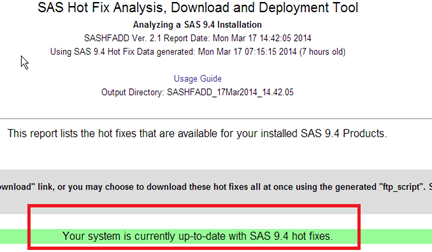*************Update End********

The followings are some quick plays and I will report more after more research:

## 1. Create a List

It’s easy to create a list:

data _null_;
a = ['apple', 'orange', 'banana'];
put a;
run;

the output in Log window: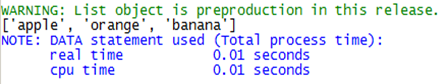You can also transfer a string to a list:

data _null_;
a = ‘SAS94′
b =list(a)
put b;
run;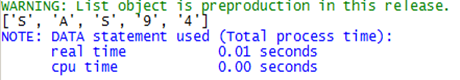## 2. Slice a List

Slicing a list is also pretty straightforward, like in R and Python:

data _null_;
a = ['apple', 'orange', 'banana'];
b = a;
c = a[:-1];
d = a[1:2];
put a;
put b;
put c;
put d;
run;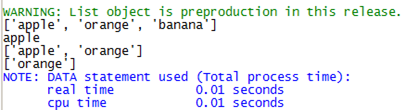## 3. List is Immutable in SAS!?

I felt much confortable to play list operations in SAS but a weird thing just happened. I tried to change a value in a list:

data _null_;
a = ['apple', 'orange', 'banana'];
a = ‘Kivi’;
put a;
run;

Unexpectedly, I got an error: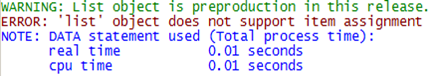hhh, I need to create a new list to hold such modification? This is funny.

Based on my quick exploration, the list object in SAS is pretty intuitive from a programmers’ point of view. But since it’s undocumented and I don’t know how long it will stay in “preproduction” phase,  just be careful to implement it in your production work.

Personally I feel very exciting to “hack” such wonderful list features in SAS 9.4. If well implemented, it will easily beat R and Python (which claim themselves supporting rich data types and objects) as a scripting language for SAS programmers. I will keep update in this page.

To perform data analysis efficiently, I need a full stack programming language rather than frequently switching from one language to another. That means — this language can hold large quantity of data, manipulate data promptly and easily (e.g. if-then-else; iteration), connect to various data sources such as relational database and Hadoop, apply some statistical models, and report result as graph, table or web. SAS is famous for its capacity to realize such a data cycle, as long as you are willing to pay the annual license fee.
SAS’s long-standing competitor, R, still keeps growing. However, in the past years, the Python community has launched a crazy movement to port R’s jewels and ideas to Python, which resulted in a few solid applications such as pandas and ggplot. With the rapid accumulation of the data-related tools in Python, I feel more comfortable to work with data in Python than R, because I have a bias that Python’s interpreter is more steady than R’s while dealing with data, and sometimes I just want to escape from R’s idiosyncratic syntax such as `x<-4` or `foo.bar.2000=10`.

Actually there is no competition between SAS and R at all: these two dwell in two parallel universes and rely on distinctive ecosystems. SAS, Python, Bash and Perl process data row-wise, which means they input and output data line by line. R, Matlab, SAS/IML, Python/pandas and SQL manipulate data column-wise. The size of data for row-wise packages such as SAS are hard-disk-bound at the cost of low speed due to hard disk. On the contrary, the column-wise packages including R are memory-bound given the much faster speed brought by memory.
Let’s go back to the comparison between SAS and Python. For most parts I am familiar with in SAS, I can find the equivalent modules in Python. I create a table below to list the similar components between SAS and Python.
SASPython
DATA stepcore Python
SAS/STATStatsModels
SAS/Graphmatplotlib
SAS Statistical Graphicsggplot
PROC SQLsqlite3
SAS/IMLNumPy
SAS Windowing EnvironmentQt Console for iPython
SAS StudioIPython notebook
SAS In-Memory Analytics for HadoopSpark with Python
This week SAS announced some promising products. Interesting, they can be traced to some of the Python’s similar implementations. For example, SAS Studio, a fancy web-based IDE with the feature of code completion, opens an HTML server at local machine and uses a browser to do coding, which is amazingly similar to iPython notebook. Another example is SAS In-Memory Analytics for Hadoop. Given that the old MapReduce path for data analysis is painfully time-consuming and complicated, aggregating memory instead of hard disk across many nodes of a Hadoop cluster is certainly faster and more interactive. Based on the same idea, Apache Spark, which fully supports Python scripting, has just been released to CDH 5.0. It will be interesting to compare Python and SAS’s in-memory ability for data analysis at the level of Hadoop.
Before there is a new killer app for R, at least for now, Python steals R’s thunder to be an open source alternative for SAS.To perform data analysis efficiently, I need a full stack programming language rather than frequently switching from one language to another. That means — this language can hold large quantity of data, manipulate data promptly and easily (e.g. if-then-else; iteration), connect to various data sources such as relational database and Hadoop, apply some statistical models, and report result as graph, table or web. SAS is famous for its capacity to realize such a data cycle, as long as you are willing to pay the annual license fee.
SAS’s long-standing competitor, R, still keeps growing. However, in the past years, the Python community has launched a crazy movement to port R’s jewels and ideas to Python, which resulted in a few solid applications such as pandas and ggplot. With the rapid accumulation of the data-related tools in Python, I feel more comfortable to work with data in Python than R, because I have a bias that Python’s interpreter is more steady than R’s while dealing with data, and sometimes I just want to escape from R’s idiosyncratic syntax such as `x<-4` or `foo.bar.2000=10`.
Let’s go back to the comparison between SAS and Python. For most parts I am familiar with in SAS, I can find the equivalent modules in Python. I create a table below to list the similar components between SAS and Python.
SASPython
DATA steppandas
SAS/STATStatsModels
SAS/Graphmatplotlib
SAS Statistical Graphicsggplot
PROC SQLsqlite3
SAS/IMLNumPy
SAS Windowing EnvironmentQt Console for iPython
SAS StudioiPython notebook
SAS In-Memory Analytics for HadoopSpark with Python
This week SAS announced some promising products. Interesting, they can be traced to some of the Python’s similar implementations. For example, SAS Studio, a fancy web-based IDE with the feature of code completion, opens an HTML server at local machine and uses a browser to do coding, which is amazingly similar to iPython notebook. Another example is SAS In-Memory Analytics for Hadoop. Given that the old MapReduce path for data analysis is painfully time-consuming and complicated, aggregating memory instead of hard disk across many nodes of a Hadoop cluster is certainly faster and more interactive. Based on the same idea, Apache Spark, which fully supports Python scripting, has just been released to CDH 5.0. It will be interesting to compare Python and SAS’s in-memory ability for data analysis at the level of Hadoop.
Before there is a new killer app for R, at least for now, Python steals R’s thunder to be an open source alternative for SAS.One trivial routine to work with PC SAS is to clear many HTML files created by using SAS, which usually occupy large hard disk space. By default, Windows does not have shell language to find the files with the same prefix as `sashtml`. I found that iPython is a convenient alternative for a shell language to conduct regular expression, and I only need a few lines of codes to realize my goal.
``%bookmark saswork c:\mydocument!cd saswork  a = !dir b = a.grep('sashtml').fields(4) !del \$b.s``• Fibonacci number
• ``#-------------------------------------------------------------------------------# Name:        Fibonacci number# Purpose:## Note:      http://en.wikipedia.org/wiki/Fibonacci_number#-------------------------------------------------------------------------------# Bad solution by recursiondef fib_rcs(n):    if n in [1, 2]:        return 1    return fib_rcs(n-1) + fib_rcs(n-2)# Good solution by iterationdef fib_itr(n):    a = *n    for i in range(2, n):        a[i] = a[i-1] + a[i-2]    return a[n-1]# Better solution by mathematicsdef fib_mth(n):    cnst = 5**0.5    phi = (1 + cnst)/2;    return int((phi**n - (1-phi)**n) / cnst)print fib_rcs(25) # cost too much timeprint fib_itr(25)print fib_mth(25)``
• Pascal’s Triangle
``#-------------------------------------------------------------------------------# Name:        Pascal's Triangle# Purpose:##             Given an index k, return the kth row of the Pascal's triangle.##             For example, given k = 3,#             Return [1,3,3,1].##             Note:#             Could you optimize your algorithm to use only O(k) extra space?##-------------------------------------------------------------------------------# Bad solution by recursiondef pascal_rcs(n):    if n == 0: return     return  + [pascal_rcs(n-1)[i] + pascal_rcs(n-1)[i-1] for i in range(1, n)] + # Good solution by iterationdef pascal_itr(n):    result =     for row in range(1, n + 1):        current =         for col in range(1, row):            current.append(result[col-1] + result[col])        result = current +     return resultprint pascal_rcs(5)print pascal_itr(5)print pascal_rcs(9) # cost too much time print pascal_itr(9) #-------------------------------------------------------------------------------# Name:        Pascal's Triangle II# Purpose:#         Given numRows, generate the first numRows of Pascal's triangle.##         For example, given numRows = 5,#         Return##         [#              ,#             [1,1],#            [1,2,1],#           [1,3,3,1],#          [1,4,6,4,1]#         ]##-------------------------------------------------------------------------------def pascal_tgl_inside(n):    result =     for row in range(1, n + 1):        yield result        current =         for col in range(1, row):            current.append(result[col-1] + result[col])        result = current + def pascal_tgl(n):    if n < 1:        return []    return list(pascal_tgl_inside(n))print pascal_tgl(8)``
• Unique Paths
``#-------------------------------------------------------------------------------# Name:        Unique Paths# Purpose:##             A robot is located at the top-left corner of a m x n grid (marked 'Start' in the diagram below).##             The robot can only move either down or right at any point in time. The robot is trying to reach the bottom-right corner of the grid (marked 'Finish' in the diagram below).##             How many possible unique paths are there?###             Above is a 3 x 7 grid. How many possible unique paths are there?##             Note: m and n will be at most 100.#-------------------------------------------------------------------------------# Bad solution by recursiondef path_rcs(m, n):    if m == 1 or n == 1:        return 1    return path_itr(m-1, n) + path_itr(m, n-1)# Good solution by iterationdef path_itr(row, col):    res = [*col]*row    for i in range(1, row):        for j in range(1, col):            res[i][j] = res[i-1][j] + res[i][j-1]    return res[row-1][col-1]print path_rcs(4, 4)print path_itr(4, 4)``
• Merge Intervals
``#-------------------------------------------------------------------------------# Name:        Merge Intervals# Purpose:##             Given a collection of intervals, merge all overlapping intervals.##             For example,#             Given [1,3],[2,6],[8,10],[15,18],#             return [1,6],[8,10],[15,18].##-------------------------------------------------------------------------------def mrg_itr(z):    if len(z) == 0:        return []    z.sort(key = lambda x: x)    carrier = z    result = [carrier]    for i in range(1, len(z)):        if carrier >= z[i]:            current = [carrier, max(carrier, z[i])]            result.pop()        else:            current = z[i]        result.append(current)        carrier = result[-1]    return resultz = [[1,4],[2,6],[8,10],[9, 12],[15,18]]print mrg_itr(z)``